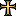# Question about the Teutonic Order

#### Ruwaard

##### Imperial Vicar of the HRE
•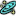•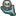•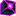•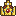•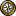•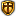•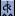•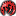•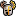•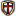•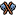•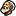•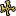•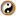•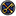•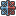•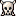•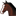•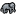•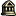•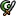•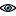•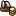•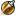•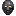•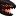•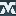•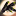•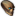•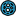•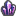•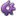•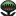•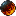•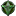•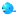••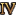•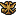•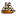•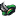•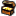•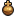•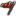•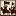•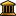•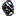••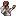•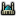I don't hate the event, but I would like to edit the event, so that it won't fire for Catholic provinces. The line I added is in red.

####################################
# Grant Land to the Teutonic Order #
####################################
province_event = {
id = 912

picture = "event_templars"

trigger = {
condition = { type = not value = { type = capital value = yes } }
condition = {
type = or
condition = { type = province value = 378 }
condition = { type = province value = 375 }
condition = { type = province value = 372 }
condition = { type = province value = 370 }
}
condition = { type = not value = { type = has_province_effect value = { heretic = yes } } }
condition = { type = not value = { type = religion value = catholic }}
condition = { type = crusade }
condition = { type = ruler_religion value = catholic }
condition = { type = ruler_piety value = 0 }
condition = {
type = not
value = {
type = title_exists
value = TEUT
}
}
condition = { type = owner condition = { type = not value = { type = trait value = sceptical } } }
condition = { type = not value = { type = year value = 1300 } }
}

mean_time_to_happen = {
months = 19
}

action_a = {
ai_chance = 90
effect = { type = create_tag value = TEUT }
effect = { type = add_regiment country = TEUT culture = teutonic_order strength = { heavy_cav = 500 heavy_inf = 1000 } }
effect = { type = ruler_piety value = 25 }
}
action_b = {
ai_chance = 10
effect = { type = ruler_piety value = -50 }
effect = { type = random chance = 33
effect = { type = add_province_effect value = heretic }
}
}
}

Does this work?

#### Veldmaarschalk

##### Very old guard
•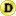•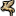••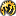••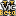•